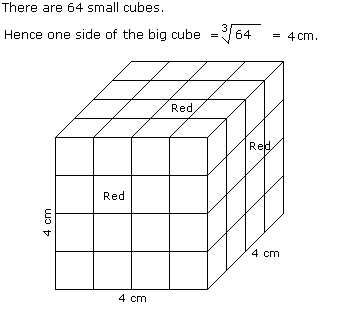# Verbal Reasoning - Cube and Cuboid - Discussion

Discussion Forum : Cube and Cuboid - Cube and Cuboid 4 (Q.No. 4)
Directions to Solve

The following questions are based on the information given below:

1. All the faces of cubes are painted with red colour.
2. The cubes is cut into 64 equal small cubes.

4.
How many small cubes are there whose two adjacent faces are coloured red ?
0
8
16
24
Explanation:Number of small cubes having two adjacent faces coloured red = (x - 2) x No. of edges

= (4 - 2) x 12

= 24

Discussion:
5 comments Page 1 of 1.

Total no. of small cubes is 64.

Cubes having two adjacent faces coloured = 64 - 8 (cubes having no coloured face) - 8 ( cubes having 3 faces coloured) = 48 cubes.

So, in my opinion, the correct answer is 48.

Hari krishna said:   7 years ago
In the question he asked two adjacent faces are coloured red, not at least two adjacent faces are coloured red, so we will count the cubes with sides coloured, in my opinion, this is definitely wrong.

Sanchita roy said:   8 years ago

So the side of each edge is 4 units and the adjacent faces on the edges is red consisting 4 cubes of 1 cubic unit. The no of faces are 6.

Multiplying no of cubes of each edge to the total no of faces gives the no of cubes having two ADJACENT faces colored red I. E, 4*6=24.

Bhavna Tayenjam said:   10 years ago
My opinion:

The two middle cubes in every edges should be counted i.e. a total of 8 middle cubes at the edges.

The cubes located at the 4 vertices of the cubes must also be counted even though they have 3 faces colored red. It still has its two adjacent faces colored.

So taking into account of these facts, the total small cubes having two adjacent faces colored red = 24+8.